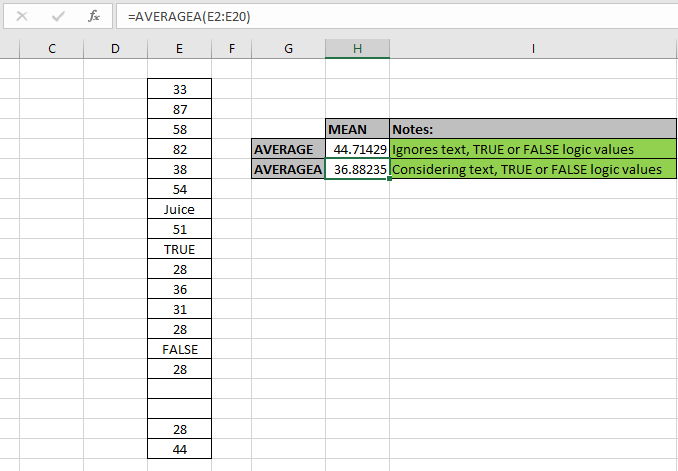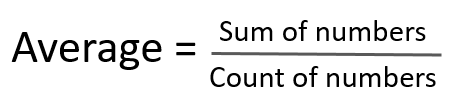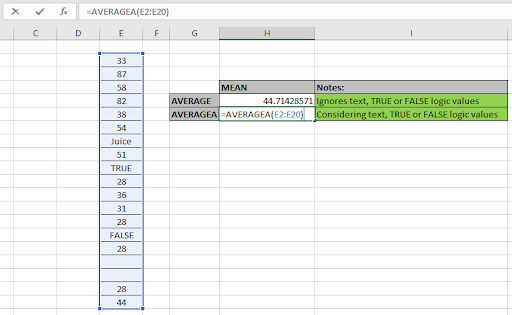# How to use the AVERGEA function in ExcelIn this article, we will learn about how to use the AVERAGEA function in Excel to calculate the Average or mean for the range of values.

What is AVERAGE or MEAN? And What type of Excel functions use it?

AVERAGEA function is a built-in function to calculate the average or mean of the range of data values provided. The average or mean for the set of values is calculated using the formula shown below.Here

1. Sum of numbers is the mathematical addition of values.
2. Count of numbers (n) is the total count of values considered.

There are newer and updated versions of AVERAGE function used for a different set of dataset values.

1. AVERAGEA function : This function is used for values considering text as 0, TRUE as 1 and FALSE as 0 and numbers in text format.
2. AVERAGE function : This function is used for values considering only numbers which are in number format.
3. AVERAGEIF function : This function takes the average of values in range applying any one condition over the corresponding or the same range.
4. AVERAGEIFS function : This function takes the average of values in range applying any multiple condition over the corresponding or the same range.

AVERAGEA function in excel returns a number which is the average or mean of the input numbers, considering logic values TRUE as 1 or FALSE as 0, text values as 0 and numbers in text format.

Syntax of AVERAGEA function:

=AVERAGEA ( Value 1, [value 2], ...)

value 1 : First value ( necessary )

value 2 : Second value ( optional ). The values can be given using cell reference.

Example:

All of these might be confusing to understand. So, let's test this formula via running it on the example shown below. Here we have some random data values to test the AVERAGEA function in Excel.

Here we have a range of values from E2:E20 cell and we need to get the average or mean for the same range using the below formula

Use the formula:

=AVERAGEA(E2:E20)See the formula in the cell. Here array value arguments to the function are given using the cell reference method. Using the above formula in the cell to get the average of values or mean of the values.The Average or mean for the random data values considering text values and Logic values is 32.88235 whereas AVERAGE function returns 44.71429 for the same data set values. This difference occurs as the value of n (count of values) in the formula changes, as in AVERAGEA case value of n is 19 (includes text & logic values) but for AVERAGE case value of n is 14 ( ignores text & Logic values ).

Here are some observational notes shown below.

Notes:

1. The function takes 255 values as maximum count.
2. If data has some particular criteria, use the AVERAGEIF function & AVERAGEIFS function.
3. The function considers some data set number as date, time, etc. So Careful using the values with date or time values.

Hope this article about How to use the AVERAGEA function in Excel is explanatory. Find more articles on statistical function formulas here. If you liked our blogs, share it with your fristarts on Facebook . And also you can follow us on Twitter and Facebook . We would love to hear from you, do let us know how we can improve, complement or innovate our work and make it better for you. Write to us at info@exceltip.com.
Related Articles

How to use the AVERAGE function in Excel : AVERAGE function is used in Excel for values considering only numbers which are in number format.

How to use the AVERAGEIF function in Excel : This function takes the average of values in range applying any one condition over the corresponding or the same range.

How to use the AVERAGEIFS function in Excel : This function takes the average of values in range applying any multiple conditions over the corresponding or the same range.

How to Use STDEV Function in Excel : Calculate the standard deviation for the data numbers in excel using the STDEV function.

How to use the VAR function in Excel : Calculate the variance for the sample data numbers in excel using the VAR function.

Popular Articles:

50 Excel Shortcuts to Increase Your Productivity | Get faster at your task. These 50 shortcuts will make you work even faster on Excel.

The VLOOKUP Function in Excel | This is one of the most used and popular functions of excel that is used to lookup value from different ranges and sheets.

COUNTIF in Excel 2016 | Count values with conditions using this amazing function. You don't need to filter your data to count specific values. Countif function is essential to prepare your dashboard.

How to Use SUMIF Function in Excel | This is another dashboard essential function. This helps you sum up values on specific conditions.

Terms and Conditions of use

The applications/code on this site are distributed as is and without warranties or liability. In no event shall the owner of the copyrights, or the authors of the applications/code be liable for any loss of profit, any problems or any damage resulting from the use or evaluation of the applications/code.